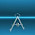## Monday, November 10, 2008

### Sudoku: Constraint Programming vs MIP

The Sudoku puzzle can be coded easily using constraint programming thanks to built-in constructs that handle the all-different constraint. Here is an example using the Microsoft Solver Foundation CSP solver:

The only difficulty was to model: for all i,j such that d[i,j]>0 require that x[i,j]=d[i,j]. I used the following formulation: Foreach[{i,I},{j,J},x[i,j]==d[i,j] | d[i,j]==0] where | is an "or" operator.

The complete model looks like:
``Model[   Parameters[Sets,I,J],  Parameters[Integers,d[I,J]],   Decisions[Integers[1,9],x[I,J]],   Constraints[     // Alternative 1: (does not work in the beta)    //FilteredForeach[{i,I},{j,J},d[i,j]>0,x[i,j]==d[i,j]],    // Alternative 2:     Foreach[{i,I},{j,J},x[i,j]==d[i,j] | d[i,j]==0],     Foreach[{i,I},Unequal[Foreach[{j,J},x[i,j]]]],    Foreach[{j,J},Unequal[Foreach[{i,I},x[i,j]]]],      Foreach[{ib,3},        Foreach[{jb,3},            Unequal[Foreach[{i,ib*3,ib*3+3},{j,jb*3,jb*3+3},x[i,j]]]         ]    ]  ] ]``

The above sudoku can also be coded in GAMS:

``\$ontext  Sudoku solver  September 2005   Code written by:     Paul van der Eijk     GAMS Development Corporation     1217 Potomac Street NW     Washington DC, 20007     Tel: (202) 342-0180 Fax: (202) 342-0181     Email: paul@gams.com     WWW: http://www.gams.com   Based on Latin Square example in GAMS model library.   Adapted to solve a Sudoku problem by:     Sherman Robinson     Economics Department     University of Sussex     Brighton BN1 9SN     UK     email: sherman.robinson@sussex.ac.uk  \$offtext sets r  rows    / r1 * r9/ c  columns / c1 * c9/ v  values  / v1 * v9/ sc sub-col /sc1 * sc3/ sr sub-row /sr1 * sr3/ scs(sc, c) /sc1.(c1*c3), sc2.(c4*c6), sc3.(c7*c9)/ srs(sr, r) /sr1.(r1*r3), sr2.(r4*r6), sr3.(r7*r9)/; table problem(r,c)      c1  c2  c3  c4  c5  c6  c7  c8  c9r1     5   3           7r2     6           1   9   5r3         9   8                   6r4     8               6               3r5     4           8       3           1r6     7               2               6r7         6                   2   8r8                 4   1   9           5r9                     8           7   9;display problem; Parameter  value(v) "Values";value(v) = ord(v) ; binary variable x(r,c,v);variable w;equations  eq1(r,c)        "exactly one value for each cell"  eq2(c, v)       "columns entries have to be unique"  eq3(r, v)       "row entries have to be unique"  eq4(sr, sc, v)  "sub-squares have to be unique"  nobj            "definition of objective - anything"; eq1(r, c)..   sum(v, x(r, c, v)) =E= 1;eq2(c, v)..   sum(r, x(r, c, v)) =E= 1;eq3(r, v)..   sum(c, x(r, c, v)) =E= 1;eq4(sr, sc, v).. sum((c,r)\$(scs(sc, c) and srs(sr, r)), x(r, c, v)) =E= 1; nobj..      w =E= sum((r, c, v), x(r, c, v)); *SR Fix values in the problemx.l(r,c,v)\$(problem(r,c) = value(v)) = 1 ;x.fx(r,c,v)\$(x.l(r,c,v) = 1)         = 1 ; option limrow=1000, limcol=0;option mip=cplex;model sudoku /eq1, eq2, eq3, eq4, nobj/;solve sudoku minimizing w using mip; parameter  solution(r,c)   "Display of results";loop(v,  solution(r, c)\$(x.l(r, c, v)) = Ord(v););option solution:0:1:1;display solution;``

I wrote an Excel based GUI for this model as an example, see http://www.amsterdamoptimization.com/packaging.html.

1.v1 of MS Solver Foundation will be out this friday.

A better way to formulate x.fx(i,j)\$(d(i,j)>0)=d(i,j)) in Microsoft's OML is

FilteredForeach[{i,I},{j,J},d[i,j]>0,x[i,j]==d[i,j]]

(this only will work with the release version, not the beta).

2.See http://blogs.msdn.com/lengningliu/ for more Sudoku related Solver Foundation code.

3.Erwin -

I put your blog on the Expert System Consulting Group blog at http://exscg.blogspot.com/ - another BlogSpot site but one devoted to the more technical side of things. If you would like to blog there as well (comments seem to be ignored on most blogs of this nature) let me know and I'll put you on the list.

BTW, I really enjoyed this since I didn't know about the Microsoft Solver Foundation tool. Really nice.

SDG
jco

4.Warning: I am not really an expert in Constraint Programming. Just use it here when it seems easier compared to a MIP model.